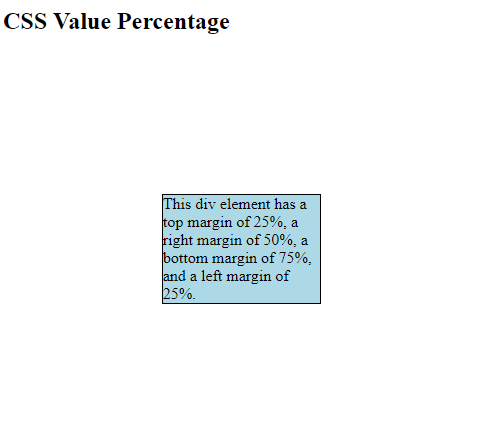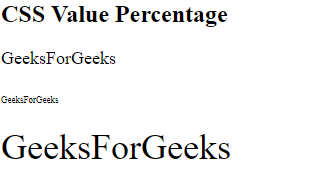# CSS | Percentage Value

• Last Updated : 03 Jun, 2020

It represents by <percentage > takes a number as a parameter. It is used to define the relative size to the parent size. With the help of this, you can adjust all the HTML-CSS elements. In CSS, many properties that take a percentage as parameters such as padding, width, height, margin, and font-size.

Syntax:

`<Percentage>`

It takes a number as parameter followed by percentage sign(%).

The number can be positive and negative but some properties do not accept negative percentages.

Note: No space between number and percentage sign(%) is acceptable.

Example 1:

 ```<``html``>`` ` `<``head``>``    ``<``style``>``        ``div {``            ``border: 1px solid black;``            ``margin: 25% 50% 75% 25%;``            ``background-color: lightblue;``        ``}``    `````` ` `<``body``>``    ``<``h2``>CSS Value Percentage`` ` `    ``<``div``>``        ``This div element has a top margin``        ``of 25%, a right margin of 50%, a ``        ``bottom margin of 75%, and a left ``        ``margin of 25%.``    ````    ``<``hr` `/>```` ` ``

Output:Example 2:

 ```<``html``>`` ` `<``head``>``    ``<``title``>``        ``CSS | Percentage Value``    `````` ` `<``body``>``    ``<``h2``>CSS Value Percentage`` ` `    ````    ``<``div` `style``=``"font-size: 18px;"``>`` ` `        ``<``p``>GeeksForGeeks`` ` `        ````        ``<``p``><``span` `style``=``"font-size: 50%;"``>``                ``GeeksForGeeks``            ````        ```` ` `        ````        ``<``p``><``span` `style``=``"font-size: 200%;"``>``                ``GeeksForGeeks``            ````        ````    ```` ` `    ``<``hr` `/>```` ` ``

Output:My Personal Notes arrow_drop_up